Equate the function and its derivatives to the initial conditions and place them in a vector named z(x).
Now, we use the initial conditions to form the linear system in for the unknowns c1, c2 ,c3.
Display the gen. soln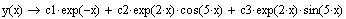Define the general solution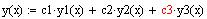c) To find a particular solution, we first form the general solution
The Wronskian is never zero since e 3x is never zero. Thus, the solutions are linearly independent.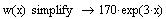The above results can be simplified using the simplify command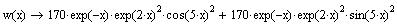Compute the Wronskian
Define the Wronskian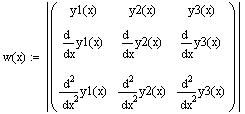b) To show that the three solutions are linearly independent, we first define the Wronskian of the solutions then compute it
This way you take care of the definition of y1(x) wither the root is real or complex. Similar definitions can be given to the other solutions.
Left-hand side is zero which is equal to the right-hand side.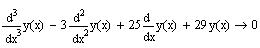We can verify our solution by substituting y(x) and its derivatives in the diff. eqn
Display the particular soln.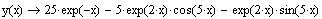You must redefine the general solution again.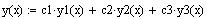Use the values returned in s to define the constants c1, c2 and c3.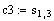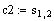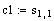The particular solution is given by
Use the solve command to find c1, c2 and c3. Name the solution s.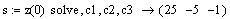Evaluate the function and its derivatives ant x = 0.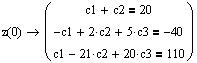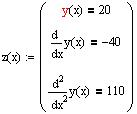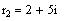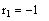We can display the roots using the subscripts of r as follows:
Use Mathcad solve command to find the roots of the char. poly and name the solution r. Mathcad will return the roots in a 3 x 1 vector.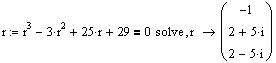Define the characteristic poly.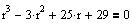a)
Force Mathcad to start the subscription from 1 rather than 0.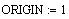The solution of this quiz will illustrate how to use Mathcad to answer the above questions
Solution
Given the differential equation y'' - 3y'' + 25y' + 29y = 0,

a) find three linearly independent solutions to the diff. eqn.

b) show that the three solutions are linearly independent. Justify your answer.

c) find a particular solution satisfying the initial conditions:

y(0) = 20, y'(0) = -40, and y''(0) = 110
This worksheet should be used as guide for solving the computer project
Computer Project Guide
Aug. 12, 2003
Math 260- 023(A Farhat)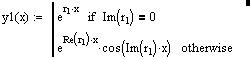You can make your definitions of the solution more general by creating the definitions in the following manner
Remark: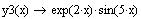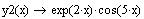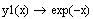Display the solutions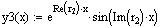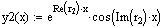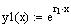Use the above roots to construct the three solutions: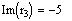and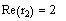The commands Re and Im are used to refer to the real and imaginary parts of a complex number. In our example,
Remark: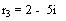and